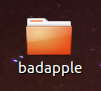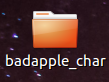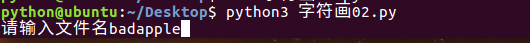## 二 ）详细步骤：

### 一 .提取视频所有帧的图片

重启大法好，重启吧。。。一重启。。。果然，还是没有好！桌面根本显示不出来东西！只有下面一个任务栏，我就在缓慢的电脑反应下 打开此电脑 找到桌面文件夹拖着缓慢的鼠标划啊划选中所有图片终于删掉了。。。。世界清净了。。。这里就告诉大家千万要改一下路径把图片导到一个文件夹里，不然直接导出到桌面，别来找我。。。找谁都不好使！）

ps:提取视频图片的步骤不做演示，网上很多教程，非常简单。

### 二 .修改代码,一次性处理所有图片

#### （1）RGB—>灰度值—>字符映射

def get_char(r,g,b,alpha = 256):

"""将256灰度映射到70个字符上"""

if alpha == 0:

return ' '
length = len(ascii_char)
"""gray = int(0.2126 * r + 0.7152*g + 0.0722 * b)

unit = (256 + 1.0)/length
return ascii_char[int(gray/unit)]
"""
gray = int(0.2126 * r + 0.7152*g + 0.0722 * b)

return ascii_char[int(gray/256*length)]


#### （2）命令行参数解析

def parser_image():

#命令行输入参数处理
parser = argparse.ArgumentParser()

parser.add_argument('--width',type = int, default = 350) #输出字符画宽
parser.add_argument('--height',type = int, default = 150)#输出字符画高

#获取参数
args = parser.parse_args()
return args


#### （3）图片处理

def handle_image(file_name, output_name, old_folder_name, new_folder_name):
args = parser_image()

IMG = old_folder_name + "/" + file_name # 将一个路径路径  源文件夹/文件名 赋值给IMG

WIDTH = args.width

HEIGHT = args.height

OUTPUT = output_name
im = Image.open(IMG) #打开IMG路径下的文件
im = im.resize((WIDTH,HEIGHT),Image.NEAREST) #给图片重新设置大小

txt = ""  #给txt一个空文本
for i in range(HEIGHT):   #逐行逐列处理图片
for j in range(WIDTH):
txt += get_char(*im.getpixel((j,i)))#获取（j,x）坐标的像素值并传给get_char()转换为对应的字符
txt += '\n' #每处理一行加上换行符
print(txt) #输出到终端
#字符画输出到文本
#if OUTPUT:
with open(new_folder_name + "/" + OUTPUT,'w') as f:  #通过 with上下文管理器 以只写方式打开 指定路径下的文件
f.write(txt)   #写入txt文本 完成后with上下文管理器会自动帮我们关闭文件
"""else:
with open("output.txt",'w') as f:
f.write(txt)"""



#### （4）主函数部分

def main():

old_folder_name = input("请输入文件名") #输入我们要处理的文件夹名称

try:
new_folder_name = old_folder_name + "_char" #给新文件夹一个名字
os.mkdir(new_folder_name)#创建new_folder_name文件夹用来写入我们的字符画
except:
pass
file_names = os.listdir(old_folder_name)  #通过os.listdir()方法获取源文件夹下的所有文件并以列表形式存储
file_names.sort()  #os.listdir()方法获取的文件名是无序的所以我们通过sort()进行一个简单的排序。
po = multiprocessing.Pool(5)  #创建一个进程池，这个进程池最多能同时容纳5个进程
#print(file_names)
for file_name in file_names: #按顺序遍历文件名
po.apply_async(handle_image, args=(file_name, file_name.replace("jpg","txt"), old_folder_name, new_folder_name))#将handle_image函数扔到进程池里面等待进程池处理
po.close()
po.join() #等待进程池里面的所有内容执行完毕
end = time.time() #这里我是为了记录代码执行时间
print("Running time: %s seconds"%(end - start))


#### （5）导入相关模块

import os #文件读写模块
import multiprocessing  #多进程
from PIL import Image
import argparse
import time   #time模块


#### （6）其他注意事项

import os
import multiprocessing
from PIL import Image
import argparse
import time
start = time.time()
ascii_char = list("\$@B%8&WM#*oahkbdpqwmZO0QLCJUYXzcvunxrjft/\|()1{}[]?-_+~<>i!lI;:,\"^'. ")

def get_char(r,g,b,alpha = 256):

"""将256灰度映射到70个字符上"""

if alpha == 0:

return ' '
length = len(ascii_char)
"""gray = int(0.2126 * r + 0.7152*g + 0.0722 * b)

unit = (256 + 1.0)/length
return ascii_char[int(gray/unit)]
"""
gray = int(0.2126 * r + 0.7152*g + 0.0722 * b)

return ascii_char[int(gray/256*length)]
def parser_image():

#命令行输入参数处理
parser = argparse.ArgumentParser()

parser.add_argument('--width',type = int, default = 350) #输出字符画宽
parser.add_argument('--height',type = int, default = 150)#输出字符画高

#获取参数
args = parser.parse_args()
return args

def handle_image(file_name, output_name, old_folder_name, new_folder_name):
args = parser_image()

IMG = old_folder_name + "/" + file_name

WIDTH = args.width

HEIGHT = args.height

OUTPUT = output_name
im = Image.open(IMG)
im = im.resize((WIDTH,HEIGHT),Image.NEAREST)

txt = ""
for i in range(HEIGHT):
for j in range(WIDTH):
txt += get_char(*im.getpixel((j,i)))
txt += '\n'
print(txt)
#字符画输出到文本
#if OUTPUT:
with open(new_folder_name + "/" + OUTPUT,'w') as f:
f.write(txt)
"""else:
with open("output.txt",'w') as f:
f.write(txt)"""

def main():

old_folder_name = input("请输入文件名")

try:
new_folder_name = old_folder_name + "_char"
os.mkdir(new_folder_name)
except:
pass
file_names = os.listdir(old_folder_name)
file_names.sort()
po = multiprocessing.Pool(5)
#print(file_names)
for file_name in file_names:
po.apply_async(handle_image, args=(file_name, file_name.replace("jpg","txt"), old_folder_name, new_folder_name))
po.close()
po.join()
end = time.time()
print("Running time: %s seconds"%(end - start))
if __name__ == "__main__":
main()

`
11-2711-2351
11-2019
10-08474
06-22
11-2386
04-14
11-21
05-04
06-1038
12-28711
02-2198
05-26
12-0583
01-162万+
05-211387
05-242291点击重新获取扫码支付余额充值